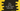# C++ program to count no of positive, negative and zero from a list of numbers## C++ program to count of positive, negative and zero from a list of user input numbers:

In this post, we will learn how to find the count of positive, negative and zero numbers from a list of user input values. Our program will ask the user to enter the total count of numbers and then it will read the numbers one by one from the user. At the end of the program, it will print the count of positive, negative and zero user has entered.

We can solve this problem in two ways:

• By using an array to store the numbers user has entered. Iterate through the array and find the counts.
• Without using an array. Read the numbers one by one from the user and find the total numbers on the fly.

I will show you both of these ways to solve this problem.

### Method 1: Using an array:

With this method, we will create one array to store the user inputs and then iterate throught the array to find the counts of each character.

``````#include <iostream>
using namespace std;

int main()
{
int positive = 0, negative = 0, zero = 0, count;

cout << "Enter the total count of numbers : " << endl;
cin >> count;

int values[count];

cout << "Enter the numbers one by one separated by space : " << endl;
for (int i = 0; i < count; i++)
{
cin >> values[i];
}

for (int i = 0; i < count; i++)
{
if (values[i] > 0)
{
positive++;
}
else if (values[i] < 0)
{
negative++;
}
else
{
zero++;
}
}

cout << "Positive : " << positive << ", Negative : " << negative << ", Zero : " << zero << endl;
}``````

### Explanation:

In this example program,

• positive, negative, zero integer variables are used to store the total positive numbers, negative numbers and zeroes we find from the user input. All are initialized with zero.
• count variable is used to store the total count of numbers that the user is going to enter. We are asking the user to enter the total numbers and storing it in count.
• Once count is entered, we are creating one integer array values of size count to hold the user entered numbers. Note that we are creating the array only after user entered the value of count. We can create one array at the start of the program with a static length like 100 but this is a better approach.
• The first for loop is used to read the user given numbers and store them in the array values.
• The second for loop is used to iterate through the array one by one and check for each value if it is positive, negative or zero. Based on its value, we are incrementing the value of positive, negative or zero variable.
• At the end of the program, we are printing the values of positive, negative and zero.

### Sample output:

If you run this program, it will print the below output:

``````Enter the total count of numbers :
5
Enter the numbers one by one separated by space :
1 2 -3 0 4
Positive : 3, Negative : 1, Zero : 1``````

### Method 2: Without using an array, counting on the fly:

We can use an array to hold the user input values if we want to store the values for future. But if we don’t want these values later on, we can simply count the positive, negative, zero values when the user enters it.

Below is the complete program:

``````#include <iostream>
using namespace std;

int main()
{
int positive = 0, negative = 0, zero = 0, count, currentValue;

cout << "Enter the total count of numbers : " << endl;
cin >> count;

cout << "Enter the numbers one by one separated by space : " << endl;
for (int i = 0; i < count; i++)
{
cin >> currentValue;

if (currentValue > 0)
{
positive++;
}
else if (currentValue < 0)
{
negative++;
}
else
{
zero++;
}
}

cout << "Positive : " << positive << ", Negative : " << negative << ", Zero : " << zero << endl;
}``````

What we changed here :

• We added one new variable currentValue that will hold the current value entered by the user and based on this value, we are incrementing the value of positive, negative or zero.
• At the end of the program, we are printing the values of positive, negative and zero similar to the previous program.

The benifit of this program is that we don’t have to create an array to store the elements. Storing any value takes memory. So, this is memory efficient than the first example.

### Output:

This program will print similar output as the above one:

``````Enter the total count of numbers :
5
Enter the numbers one by one separated by space :
1 2 -3 0 4
Positive : 3, Negative : 1, Zero : 1``````